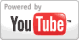Area of a Rectangle - Geometry Lesson
Grade: 6 - 12 | Math lesson on area of a rectangle. Learn the concepts of area and perimeter, as well as the formulas for the area and perimeter of a rectangle and a square. Solve problems using these formulas.Add to My DashboardGeometry### Search Videos & Games